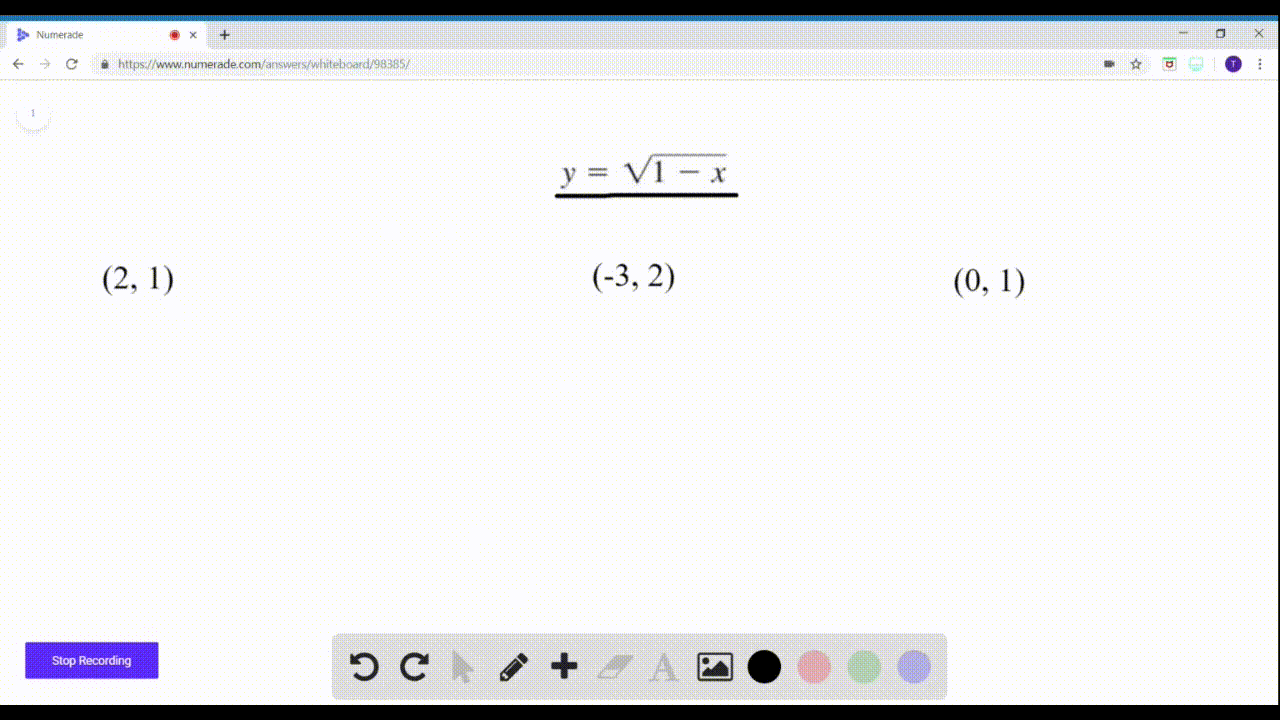🎉 The Study-to-Win Winning Ticket number has been announced! Go to your Tickets dashboard to see if you won! 🎉View Winning Ticket### $9-14=$ Points on a Graph? Determine whether the …

01:33Problem 9

# $9-14=$ Points on a Graph? Determine whether the given pointsare on the graph of the equation.$$y=3-4 x ; \quad(0,3),(4,0),(1,-1)$$

## Discussion

You must be signed in to discuss.

## Video Transcript

for this item were asked to determine whether the given points are on the graph of the equation. We're going to substitute values into this equation appropriately and check to see if it gives a true statement. Here we end up with three equals three, which is true. So 03 is on the graph. We substitute in or for X zero for why we get zero equals three. Not true. So four comma zero is not on the graph Substitute negative one for why one? Whoops. Let's make that adjustment there one. In that case, we do the calculation and we end up with negative one equals negative one. That is true, then the 0.1 negative one is on the graph.